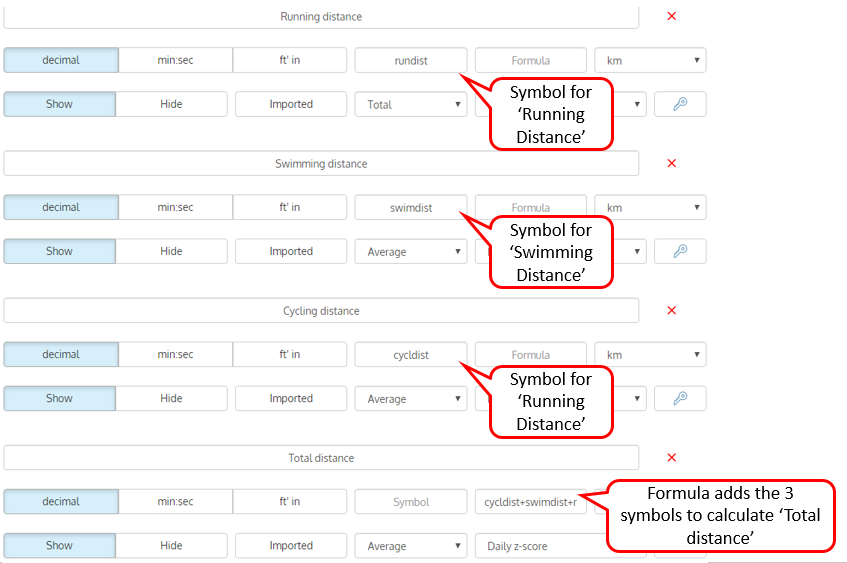With AthleteMonitoring you can combine multiple fields into a single measure and calculate your own custom metrics, using your own formulas.

For example, you can create a 'session stress index' by dividing the RPE by the session's duration, calculate a 'Total distance' from different activities performed during a session (example below), and even combine multiple data fields imported from your GPS device to generate, for example, a 'Training density' index such as playerload/distance  or playerload/duration.How to create formulas

To create a formula, you must assign a symbol to each custom field you want to include in the formula in the 'Symbol' box, and enter the formula in the 'Formula' box.  Formulas are automatically processed when sessions are saved.

Once data recorded, it's automatically integrated in the alert system and displayed on graphs, reports, dashboards.

Important : Symbols should only contain lowercase letters (eg: a symbol such as "20mSprint" will not work but "twmsprint" will). Symbols containing spaces, numbers or uppercase letters will not work.

Variables you may use in formulas

age = athlete age calculated using the birth date, according to the information stored in the athlete profile

gender = athlete gender, according to the information stored in the athlete profile

rpe =athlete age calculated using the athlete birth date, according to the information stored in the athlete profile
gender =athlete gender according to the information stored in the athlete profile

duration =duration of the session

enjoyment =enjoyment recorded for the session

The symbol of any custom field ('rundist' =running distance in the example above will return the value recorded during the session)

Math operators

- (substraction)
/(division)
* (multiplication)
^ (power)
() parenthesis

Logical operators

> (greater than)
< (less than)
<= (less than or equal)
>= (greater than or equal)
== (equal)

Mathematical functions

AthleteMonitoring use a powerful mathematical library that includes many functions that you can use in formulas. For a complete list, click here . All functions listed can be used in AthleteMonitoring formulas.

For example, if you want to round a calculated number to the 2nd decimal, you can use the 'round' function : round(symbolone/symboltwo,2)